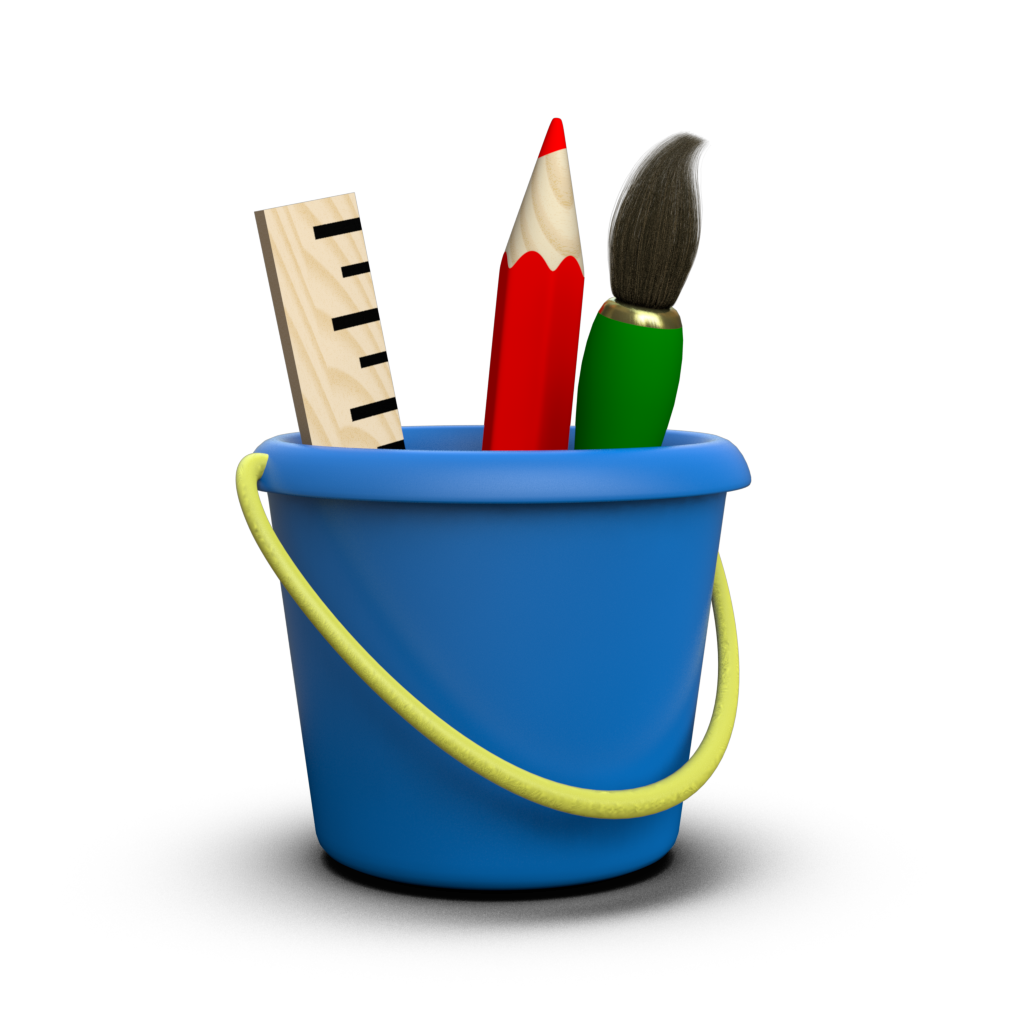# Logarithmic Scales¶

In Toyplot, individual axes supplied by toyplot.coordinates.Cartesian and toyplot.coordinates.Numberline provide mappings from data values to canvas coordinates. An important property of each axis is its scale, used to specify linear or logarithmic mappings from domain to range:

:

import numpy
x = numpy.linspace(-1000, 1000, 51)

:

import toyplot
canvas = toyplot.Canvas(width=600, height=200)

numberline = canvas.numberline(grid=(2, 1, 0, 0), scale="linear")
numberline.scatterplot(x)

numberline = canvas.numberline(grid=(2, 1, 1, 0), scale="log")
numberline.scatterplot(x);


Or in two dimensions:

:

canvas = toyplot.Canvas(width=700)

axes = canvas.cartesian(grid=(2, 2, 0, 0), xscale="linear", yscale="linear")
axes.plot(x, x, marker="o")

axes = canvas.cartesian(grid=(2, 2, 0, 1), xscale="log", yscale="linear")
axes.plot(x, x, marker="o")

axes = canvas.cartesian(grid=(2, 2, 1, 0), xscale="linear", yscale="log")
axes.plot(x, x, marker="o")

axes = canvas.cartesian(grid=(2, 2, 1, 1), xscale="log", yscale="log")
axes.plot(x, x, marker="o");


Note that Toyplot handles negative values correctly, and provides sensible results for values near zero by rendering them using a small linear region around the origin.

The scale can be specified in two ways:

• As a string - “linear”, “log” (base 10), “log10” (base 10), or “log2” (base 2).
• As a tuple - (“log”, 2), (“log”, 10).

For example, the following are all equivalent

:

canvas = toyplot.Canvas(width=600, height=300)

numberline = canvas.numberline(grid=(3,1,0), scale="log")
numberline.scatterplot(x)

numberline = canvas.numberline(grid=(3,1,1), scale="log10")
numberline.scatterplot(x)

numberline = canvas.numberline(grid=(3,1,2), scale=("log", 10))
numberline.scatterplot(x);


Of course, you are free to specify any base you like, using the tuple notation:

:

canvas = toyplot.Canvas(width=600, height=100)
numberline = canvas.numberline(scale=("log", 4))
numberline.scatterplot(x);